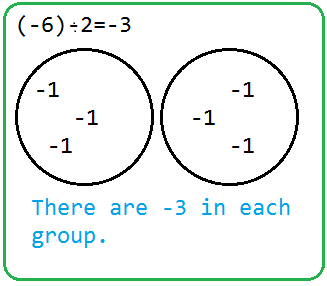## Monday, March 7, 2011

### Allison's Great Big Book Of Integers

Chapter 1: Grade 7 Integer Review
• To solve integers problems you can use number lines or integer chips
• Usually red chips represent positive numbers and blue chips represent negative numbers
• A zero pair is one negative and one positive together
A rule to remember about zero pairs are, " When subtracting something that isn't there, use a zero pair"

Here are some examples or subtracting and addition questions on integers:Chapter 2: Multiplying Integers

• When multiplying integers always remember "The Sign Rule" .
The rule is that if there is an odd numbers of negatives in the question, the answer will be a negative number.If there is an even numbers of negatives in the question, the product will be a positive. If there is only positives the answer will always be a positive.Chapter 3: Dividing Integers
• Partitive Division is when you know the total number of groups, and you are trying to find the number of items in each group.
• Quotitive Division is when you know the total items that are in each group and you're trying to find the number of groups & sharing you total with groups.QUOTITIVE DIVISIONThe sign rule of addition, subtraction and multiplication also applies to division. For example:
• 6÷2= 3
there are no negative signs therefore the answer will be a positive.
• (-6)÷(-2)= +3
there is an even number of negatives meaning the answer will be a positive.
• (-6)÷2=-3
there is an odd number of negatives so they answer will be a negative
• 6÷(-2)=-3
the same goes for this.

Chapter 4: Order of Operations with Integers